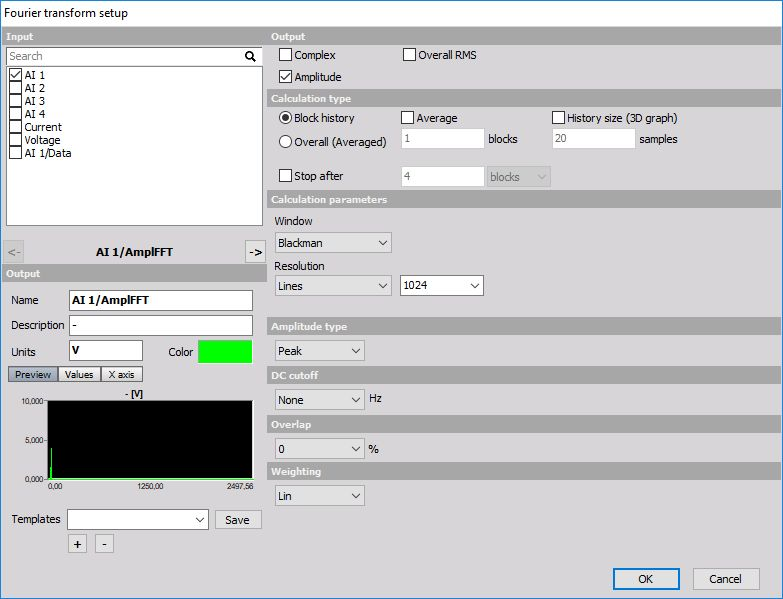# Fourier transform

When you press the Setup button on FFT analysis math item, the following setup window will open:The output of the FFT analysis could be Complex (real, imaginary), Amplitude, Phase or any combination of those.

Calculation type can be Overall (Averaged), where the result is one spectrum for the entire record. The second option is Block history, where the FFT’s are acquired shot by shot and put into the buffer. In this case, they can be observed on the 3D graph. Number of Averages can be also set. When we enter a value of n, then it will calculate the average of n spectrum and put the result in the channel. Manual history count can override the normal settings for the number of shots to be kept in the memory during measurement.

Several Calculation parameters can be also set. One of them is the Window type, which describes the FFT window. There is a good description of the usage of different window functions in the tutorial. By default, we use Blackman, because it is a good compromise between the amplitude error and width of sidebands. Number of Lines defines the size of the FFT. The more lines we choose, the more accurate the frequency will be, but also longer time is needed for the calculation. The number of samples needed is always twice of the size of resulting FFT (number which is set).

IMPORTANT: ANY number of lines can be entered, not only 2^n. We can manually enter 2000 or 10000 FFT lines. The only drawback is that the calculation will get slower if we choose strange numbers (like 12431).

Amplitude type defines what kind of amplitude is put in the channel. We have several options:

Amplitude type Units Description
Amplitude (Auto) V is the pure signal amplitude
RMS V rms is the RMS amplitude, calculated as Amplitude/sqrt(2)
Power V * V calculated as RMS value squared
PSD V * V / Hz calculated as RMS squared, divided by the line resolution and sqrt(2) - used for checking the noise
RMS SD V / sqrt(Hz) calculated as RMS value, divided by the square root of line resolution - also used for checking the nois

DC cutoff option will remove the static offset from the FFT. If it is set to None, it will output FFT as calculated, if not, it will cut the number of lines until frequency which is defined is reached.

Overlap defines how much will two FFT shots overlap between each other. 50% is enough that all the samples will have the same weight as the result independent of the window which is used.

Weighting defines which sound weighting should be used on the resulting FFT.

As all matrix channels also FFT can be best seen on the 2D graph. Block-based FFT can be also put in the 3D graph for viewing the history.